# Lightweight Airplane Design

This model shows how to use MathWorks® products to address the technical and process challenges of aircraft design using the design of a lightweight aircraft.

The design process is iterative; you will try many vehicle configurations before selecting the final one. Ideally, you perform iterations before building any hardware. The challenge is to perform the iterations quickly. Typically, different groups work on different steps of the process. Effective collaboration among these groups and the right set of tools are essential to addressing this challenge.

### Defining Vehicle Geometry

The geometry of this lightweight aircraft is from reference 1. The original design objective for this geometry is a four-seat general aviation aircraft that is safe, simple to fly, and easily maintainable with specific mission and performance constraints. For more details on these constraints, see reference 1.

Potential performance requirements for this aircraft include:

• Level cruise speed

• Acceptable rate of climb

• Acceptable stall speed

For the aircraft flight control, rate of climb is the design requirement and assumed to be greater than 2 meters per second (m/s) at 2,000 meters.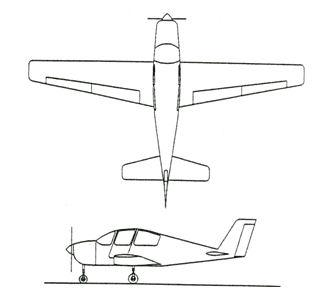Figure 1: Lightweight four-seater monoplane .

### Determining Vehicle Aerodynamic Characteristics

The aircraft's geometrical configuration determines its aerodynamic characteristics, and therefore its performance and handling qualities. Once you choose the geometric configuration, you can obtain the aerodynamic characteristics by means of:

• Analytical prediction

• Wind tunnel testing of the scaled model or a full-sized prototype

• Flight tests

While wind tunnel tests and flight tests provide high-fidelity results, they are expensive and time-consuming, because they must be performed on the actual hardware. It is best to use these methods when the aircraft's geometry is finalized. Note: Analytical prediction is a quicker and less expensive way to estimate aerodynamic characteristics in the early stages of design.

In this example, we use Digital Datcom, a popular software program, for analytical prediction. The U.S. Air Force developed it as a digital version of its Data Compendium (DATCOM). This software is publicly available.

To start, create a Digital Datcom input file that defines the geometric configuration of our aircraft and the flight conditions that we need to obtain the aerodynamic coefficients.

type asbSkyHoggDatcom.in
 $FLTCON NMACH=4.0,MACH(1)=0.1,0.2,0.3,0.35$ $FLTCON NALT=8.0,ALT(1)=1000.0,3000.0,5000.0,7000.0,9000.0, 11000.0,13000.0,15000.0$ $FLTCON NALPHA=10.,ALSCHD(1)=-16.0,-12.0,-8.0,-4.0,-2.0,0.0,2.0, ALSCHD(8)=4.0,8.0,12.0,LOOP=2.0$ $OPTINS SREF=225.8,CBARR=5.75,BLREF=41.15$ $SYNTHS XCG=7.9,ZCG=-1.4,XW=6.1,ZW=0.0,ALIW=1.1,XH=20.2, ZH=0.4,ALIH=0.0,XV=21.3,ZV=0.0,VERTUP=.TRUE.$ $BODY NX=10.0, X(1)=-4.9,0.0,3.0,6.1,9.1,13.3,20.2,23.5,25.9, R(1)=0.0,1.0,1.75,2.6,2.6,2.6,2.0,1.0,0.0$ $WGPLNF CHRDTP=4.0,SSPNE=18.7,SSPN=20.6,CHRDR=7.2,SAVSI=0.0,CHSTAT=0.25, TWISTA=-1.1,SSPNDD=0.0,DHDADI=3.0,DHDADO=3.0,TYPE=1.0$ $HTPLNF CHRDTP=2.3,SSPNE=5.7,SSPN=6.625,CHRDR=0.25,SAVSI=11.0, CHSTAT=1.0,TWISTA=0.0,TYPE=1.0$ $VTPLNF CHRDTP=2.7,SSPNE=5.0,SSPN=5.2,CHRDR=5.3,SAVSI=31.3, CHSTAT=0.25,TWISTA=0.0,TYPE=1.0$ $SYMFLP NDELTA=5.0,DELTA(1)=-20.,-10.,0.,10.,20.,PHETE=.0522, CHRDFI=1.3, CHRDFO=1.3,SPANFI=.1,SPANFO=6.0,FTYPE=1.0,CB=1.3,TC=.0225, PHETEP=.0391,NTYPE=1.$ NACA-W-4-0012 NACA-H-4-0012 NACA-V-4-0012 CASEID SKYHOGG BODY-WING-HORIZONTAL TAIL-VERTICAL TAIL CONFIG DAMP NEXT CASE 

Digital Datcom provides the vehicle's aerodynamic stability and control derivatives and coefficients at specified flight conditions. Flight control engineers can gain insight into the vehicle's performance and handling characteristics by examining stability and control derivatives. We must import this data into the MATLAB® technical computing environment for analysis. Normally, this is a manual process.

With the Aerospace Toolbox software, we can bring multiple Digital Datcom output files into the MATLAB technical computing environment with just one command. There is no need for manual input. Each Digital Datcom output is imported into the MATLAB technical computing environment as a cell array of structures, with each structure corresponding to a different Digital Datcom output file. After importing the Digital Datcom output, we can run multiple configurations through Digital Datcom and compare the results in the MATLAB technical computing environment.

alldata = datcomimport({'asbSkyHoggDatcom.out' 'asbSkyHoggDatcom_old.out'}, true, 0);

In our model, we need to check whether the vehicle is inherently stable. To do this, we can use Figure 2 to check whether the pitching moment described by the corresponding coefficient, Cm, provides a restoring moment for the aircraft. A restoring moment returns the aircraft angle of attack to zero.

In configuration 1 (Figure 2), Cm is negative for some angles of attack less than zero. This means that this configuration will not provide a restoring moment for those negative angles of attack and will not provide the flight characteristics that are desirable. Configuration 2 fixes this problem by moving the center of gravity rearward. Shifting the center of gravity produces a Cm that provides a restoring moment for all negative angles of attack.

h1 = figure; plot(alldata{2}.alpha,alldata{2}.cm(:,1),alldata{1}.alpha,alldata{1}.cm(:,1)); axis([-4 8 -0.3 0.1]); legend('Configuration 1','Configuration 2'); xlabel('Angle of Attack, \alpha (degrees)'); ylabel('Pitching Moment Coefficient, C_m'); grid;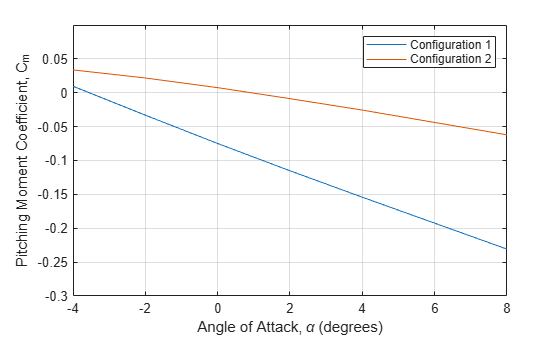Figure 2: Visual analysis of Digital Datcom pitching moment coefficients.

close (h1);

### Creating Flight Vehicle Simulation

Once we determine aerodynamic stability and control derivatives, we can build an open-loop plant model to evaluate the aircraft longitudinal dynamics. Once the model is complete, we can show it to colleagues, including those who do not have Simulink® software, by using Simulink® Report Generator™ software to export the model to a Web view. A Web view is an interactive HTML replica of the model that lets you navigate model hierarchy and check the properties of subsystems, blocks, and signals.

A typical plant model includes the following components:

• Equations of motion: Calculate vehicle position and attitude from forces and moments.

• Forces and moments: Calculate aerodynamic, gravity, and thrust forces and moments.

• Actuator positions: Calculate displacements based on actuator commands.

• Environment: Include environmental effects of wind disturbances, gravity, and atmosphere.

• Sensors: Model the behavior of the measurement devices.

We can implement most of this functionality using Aerospace Blockset™ blocks. This model highlights subsystems containing Aerospace Blockset blocks in orange. It highlights Aerospace Blockset blocks in red.

open_system('asbSkyHogg'); snapshotModel('asbSkyHogg');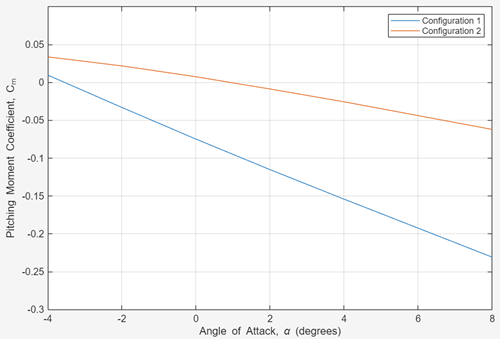Figure 3: Top Level of Lightweight Aircraft Model

We begin by building a plant model using a 3DOF block from the Equations of Motion library in the Aerospace Blockset library (Figure 4). This model helps us determine whether the flight vehicle is longitudinally stable and controllable. We design our subsystem to have the same interface as a six degrees-of-freedom (DOF) version. When we are satisfied with three DOF performance, stability, and controllability, we can implement the six DOF version, iterating on the other control surface geometries until we achieve the desired behavior from the aircraft.

open_system('asbSkyHogg/Vehicle System Model/Vehicle/3DOF to 6DOF'); snapshotModel('asbSkyHogg/Vehicle System Model/Vehicle/3DOF to 6DOF');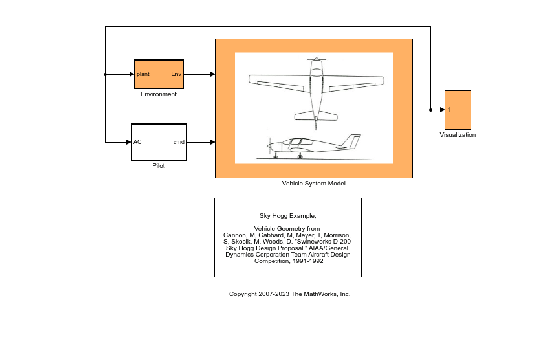Figure 4: Equations of Motion implemented using 3DoF Euler block from the Aerospace Blockset library.

To calculate the aerodynamic forces and moments acting on our vehicle, we use a Digital Datcom Forces and Moments block from the Aerospace Blockset library (Figure 5). This block uses a structure that Aerospace Toolbox creates when it imports aerodynamic coefficients from Digital Datcom.

For some Digital Datcom cases, dynamic derivative have values for only the first angle of attack. The missing data points can be filled with the values for the first angle of attack, since these derivatives are independent of angle of attack. To see example code of how to fill in missing data in Digital Datcom data points, you can examine the asbPrepDatcom function.

open_system('asbSkyHogg/Vehicle System Model/Vehicle/Aerodynamics'); snapshotModel('asbSkyHogg/Vehicle System Model/Vehicle/Aerodynamics');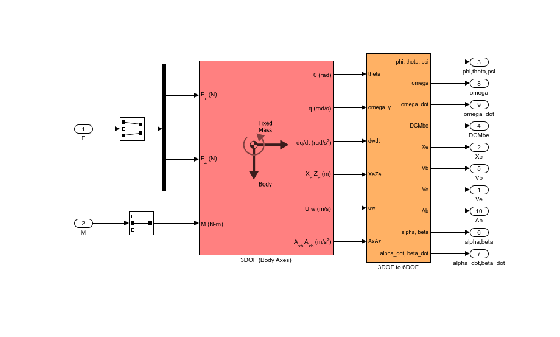Figure 5: Aerodynamic Forces and Moments implemented in part with the Aerospace Blockset Digital Datcom Forces and Moment block.

We also use Aerospace Blockset blocks to create actuator, sensor, and environment models (Figures 6, 7, and 8, respectively). Note: In addition to creating the following parts of the model, we use standard Aerospace Blockset blocks to ensure that we convert from body axes to wind axes and back correctly.

open_system('asbSkyHogg/Vehicle System Model/Vehicle/AirframeActuators'); snapshotModel('asbSkyHogg/Vehicle System Model/Vehicle/AirframeActuators');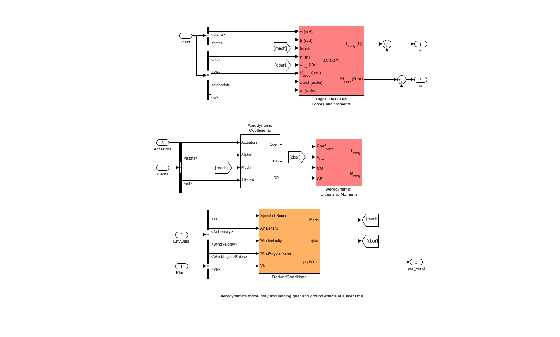Figure 6: Implementation of actuator models using Aerospace Blockset blocks.

open_system('asbSkyHogg/Vehicle System Model/Flight Sensors'); snapshotModel('asbSkyHogg/Vehicle System Model/Flight Sensors');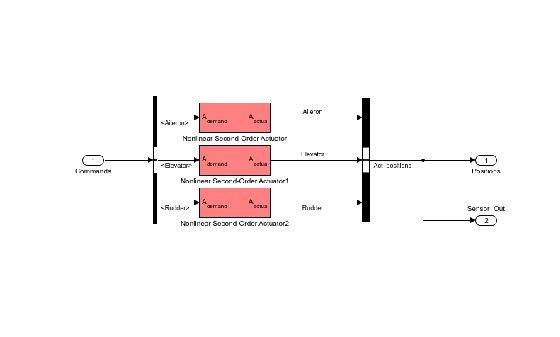Figure 7: Implementation of flight sensor model using Aerospace Blockset blocks.

open_system('asbSkyHogg/Environment'); snapshotModel('asbSkyHogg/Environment');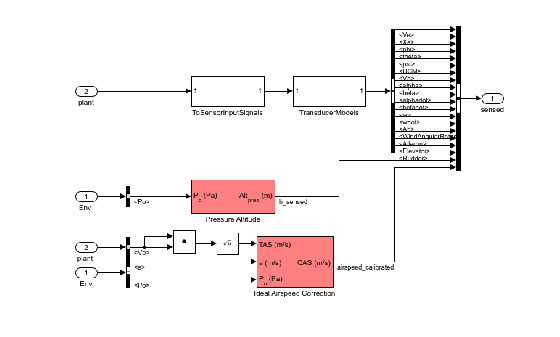Figure 8: Environmental effects of wind, atmosphere, and gravity using Aerospace Blockset blocks.

### Designing Flight Control Laws

Once we have created the Simulink plant model, we design a longitudinal controller that commands elevator position to control altitude. The traditional two-loop feedback control structure chosen for this design (Figure 9) has an outer loop for controlling altitude (compensator C1 in yellow) and an inner loop for controlling pitch angle (compensator C2 in blue). Figure 10 shows the corresponding controller configuration in our Simulink model.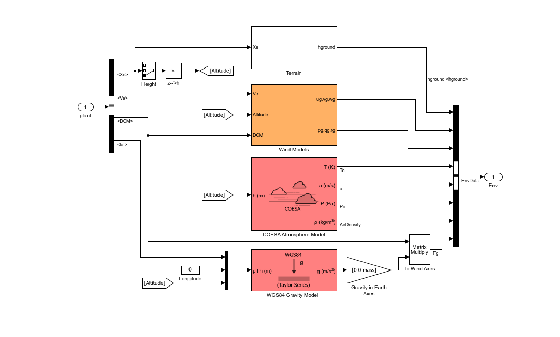Figure 9: Structure of the longitudinal controller.

open_system('asbSkyHogg/Vehicle System Model/Avionics/Autopilot'); snapshotModel('asbSkyHogg/Vehicle System Model/Avionics/Autopilot');Figure 10: Longitudinal controller in Simulink model.

With Simulink® Control Design™ software, we can tune the controllers directly in Simulink using a range of tools and techniques.

• Two controller blocks

• Closed-loop input or altitude command

• Closed-loop output signals or sensed altitude

Using this information, Simulink Control Design software automatically computes linear approximations of the model and identifies feedback loops to be used in the design. To design the controllers for the inner and outer loops, we use root locus and bode plots for the open loops and a step response plot for the closed-loop response (Figure 11).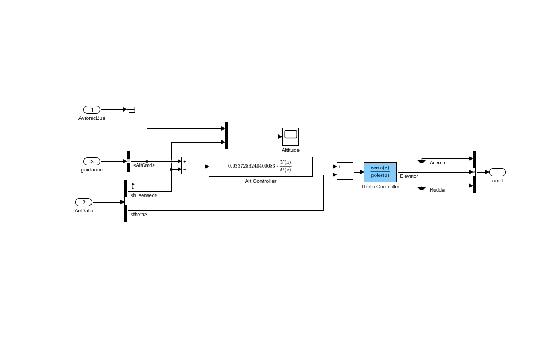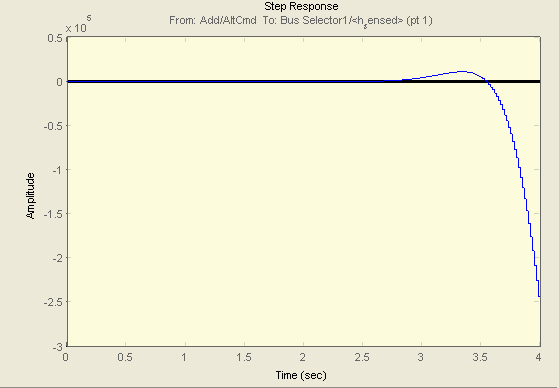Figure 11: Design plots before controller tuning.

We then interactively tune the compensators for the inner and outer loops using these plots. Because the plots update in real time as we tune the compensators, we can see the coupling effects that these changes have on other loops and on the closed-loop response.

To make the multi-loop design more systematic, we use a sequential loop closure technique. This technique lets us incrementally take into account the dynamics of the other loops during the design process. With Simulink Control Design, we configure the inner loop to have an additional loop opening at the output of the outer loop controller (C1 in Figure 12). This approach decouples the inner loop from the outer loop and simplifies the inner-loop controller design. After designing the inner loop, we design the outer loop controller. Figure 13 shows the resulting tuned compensator design at the final trimmed operating point.Figure 12: Block diagram of inner loop, isolated by configuring an additional loop opening.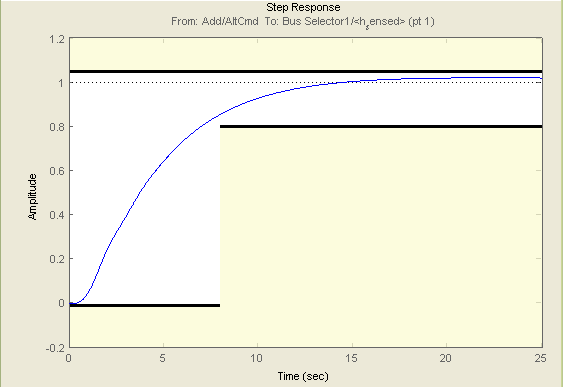Figure 13: Design plots at trim condition after controller tuning.

You can tune the controller in Simulink Control Design software in several ways. For example:

• You can use a graphical approach, and interactively move controller gain, poles, and zeros until you get a satisfactory response (Figure 13).

• You can use Simulink® Design Optimization™ software within Simulink Control Design software to tune the controller automatically.

After you specify frequency domain requirements, such as gain margin and phase margin and time domain requirements, Simulink Design Optimization software automatically tunes controller parameters to satisfy those requirements. Once we have developed an acceptable controller design, the control blocks in the Simulink model are automatically updated. See the examples Getting Started with the Control System Designer (Control System Toolbox) in Control Systems Toolbox examples and Tune Simulink Blocks Using Compensator Editor (Simulink Control Design) in Simulink Control Design examples for more information on tuning controllers.

We can now run our nonlinear simulation with flight control logic and check that the controller performance is acceptable. Figure 15 shows the results from a closed-loop simulation of our nonlinear Simulink model for a requested altitude increase from 2,000 meters to 2,050 meters starting from a trimmed operating point. Although a pilot requests a step change in altitude, the actual controller altitude request rate is limited to provide a comfortable and safe ride for the passengers.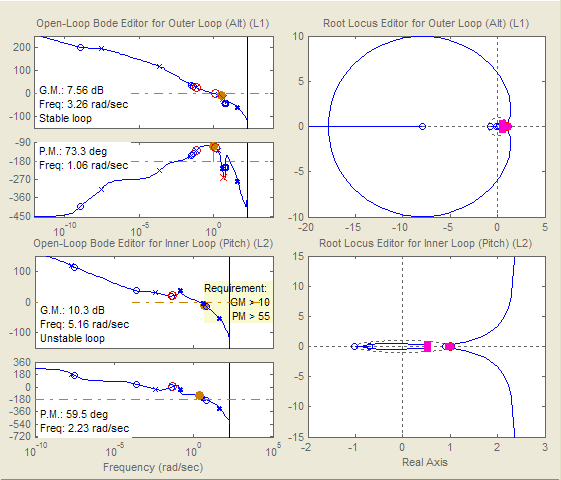Figure 14: The final check is to run nonlinear simulation with our controller design and check that altitude (purple) tracks altitude request (yellow) in the stable and acceptable fashion.

We can now use these simulation results to determine whether our aircraft design meets its performance requirements. The requirement called for the climb rate to be above 2 m/s. As we can see, the aircraft climbed from 2,000 to 2,050 meters in less than 20 seconds, providing a climb rate higher than 2.5 m/s. Therefore, this particular geometric configuration and controller design meets our performance requirements.

In addition to traditional time plots, we can visualize simulation results using the Aerospace Blockset interface to FlightGear (Figure 15).Figure 15: Visualizing simulation results using the Aerospace Blockset interface to FlightGear.

We can also use the Aerospace Toolbox interface to FlightGear to play back MATLAB data using either simulation results or actual flight test data.

### Completing the Design Process

The next steps involve

1. Building a hardware-in-the-loop system to test real-time performance.

2. Building the actual vehicle hardware and software.

3. Conducting the flight test.

4. Analyzing and visualizing the flight test data.

Because these steps are not the focus of this example, we do not describe them here. Instead, we simply mention that they can all be streamlined and simplified using the appropriate tools, such as Embedded Coder®, Simulink® Real-Time™, and Aerospace Toolbox software.

### Summary

In this example we showed how to:

• Use Digital Datcom and Aerospace Toolbox software to rapidly develop the initial design of your flight vehicle and evaluate different geometric configurations.

• Use Simulink and Aerospace Blockset software to rapidly create a flight simulation of your vehicle.

• Use Simulink Control Design software to design flight control laws.

This approach enables you to determine the optimal geometrical configuration of your vehicle and estimate its performance and handling qualities well before any hardware is built, reducing design costs and eliminating errors. In addition, using a single tool chain helps facilitate communication among different groups and accelerates design time.

### References

 Cannon, M, Gabbard, M, Meyer, T, Morrison, S, Skocik, M, Woods, D. "Swineworks D-200 Sky Hogg Design Proposal." AIAA®/General Dynamics Corporation Team Aircraft Design Competition, 1991-1992.

 Turvesky, A., Gage, S., and Buhr, C., "Accelerating Flight Vehicle Design", MATLAB® Digest, January 2007.

 Turvesky, A., Gage, S., and Buhr, C., "Model-based Design of a New Lightweight Aircraft", AIAA paper 2007-6371, AIAA Modeling and Simulation Technologies Conference and Exhibit, Hilton Head, South Carolina, Aug. 20-23, 2007.